Saltire Software NewsletterBack to School with the new version of Math Illustrations\$15 off coupon for Personal Copy of Math IllustrationsDraw precise mathematical diagrams with less hassle.Use coupon code B2SSPECIAL hereOffer good through 9/30/2016
This month, we'll show you how to use the new Point Trace feature to illustrate the pantograph, a simple machine which can be used to make scaled copies of curves.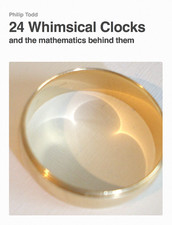Beginning later this month, look into your inbox for the first installment of a serialization of our revolutionary interactive mathematical ebook 24 Whimsical Clocks.

For a pre-taste, you can see the first clock, here

How to...draw a pantograph with Math Illustrations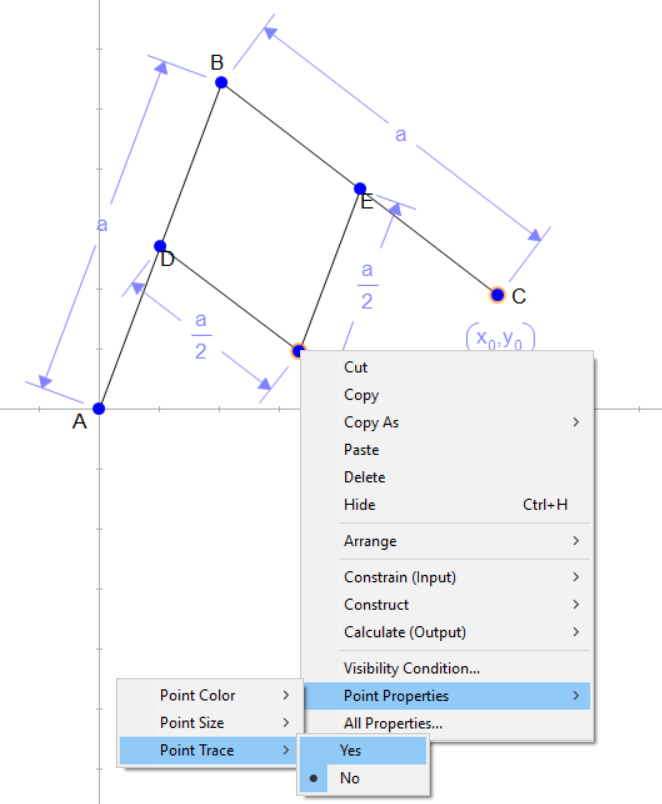Pantograph is a mechanism which creates a half or double scaled version of whatever you trace.

Here is how to model it in Geometry Expressions:
• Draw lines AB, BC with A at the origin.
• Create D,E, the midpoints of AB and BC.
• Draw lines DF and FE.
• Constrain AB and BC to be lengtha
• Constrain DF and EF to be lengtha/2.
• Constrain the coordinates of C to be (x0,y0).
• In the Variables Panel, lock variable a.
• Now select C and F and from the right click menu do Point Properties / Point Trace / Yes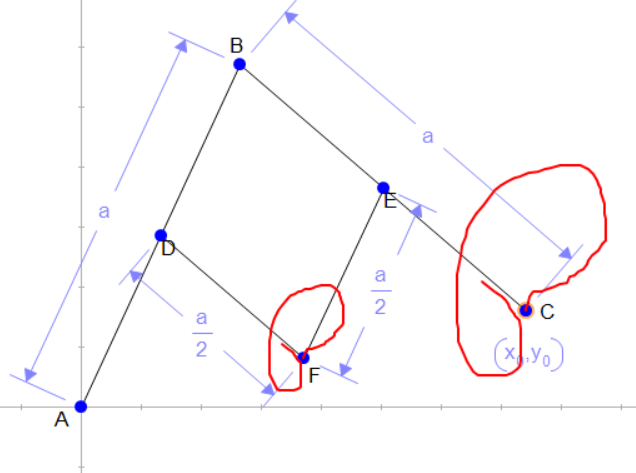Now you can drag C and you will see the trace left by both C and F

Point Traces are available in Math Illustrations v. 3.1  (and Geometry Expressions 3.3 and Mechanical Expressions 1.1).

Here is an app generated by Geometry Expressions.
 Saltire Software NewsletterProblem of the Month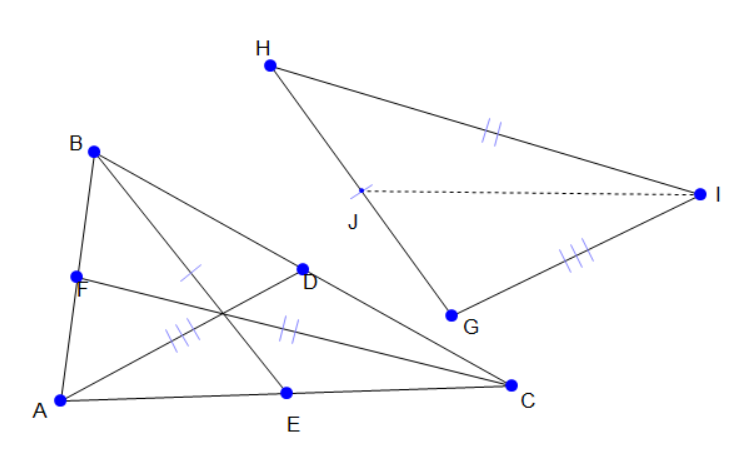Given a triangle ABC, let its medians be AD, BE and CF.

Construct a new triangle GHI whose sides have the lengths of the medians of the original triangle.

Let IJ be a median of GHI.

What is the relationship between the length of the median IJ and the lengths of the sides of the original triangle ABC?

An app which lets you explore this is here.A Solution to June's Problem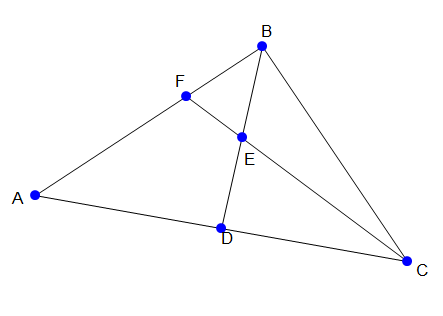Show that the line joining the midpoint of a median to a vertex of the triangle trisects the side opposite the vertex considered.

In the diagram, D is the midpoint of AC, E is the midpoint of BD.

You are asked to show that BF is 1/3 of AB.

An app which lets you explore this ishere.

Using Geometry Expressions this is an easy result to establish.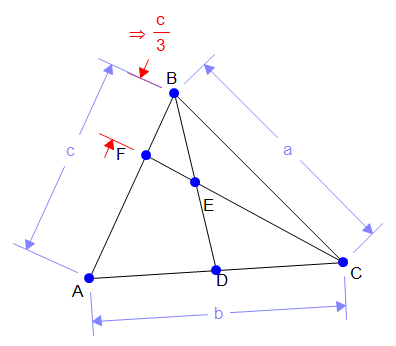Just constrain the triangle by its side lengths, create midpoint D, then midpoint E, draw CF and constrain E to lie on it.  Now measure the symbolic distance BF.

You can generalize the result by putting E at proportion t along the line BD.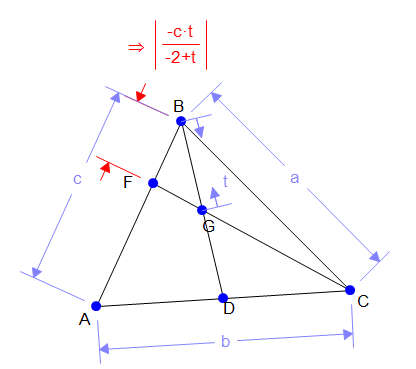Saltire Software  |  www.saltire.com

info@paviathintegratedsolution.com www.paviathintegratedsolution.com